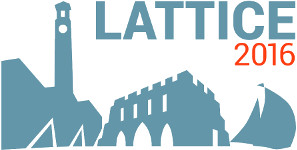#The 34th International Symposium on Lattice Field Theory (Lattice 2016)

Jul 24 – 30, 2016
Highfield Campus, University of Southampton
Europe/London timezone

## Near threshold states $D_{s0}^{*}\left(2317\right)$ and $D_{s1}\left(2460\right)$

Jul 28, 2016, 3:40 PM
20m
Building B2a Room 2065 (Highfield Campus, University of Southampton)

### Speaker

Mr Antonio Cox (University of Regensburg)

### Description

Early theoretical studies and lattice simulations predicted the charmed-strange mesons $D_{s0}^{*}(2317)$ and $D_{s1}(2460)$ to be broad states lying above the thresholds, $DK$ and $D^{*}K$, respectively. Experiments found narrow states below threshold. We present results of a high statistics $Nf=2$ study with a lattice spacing of approximately $0.071$ fm, taking explicitly into account the thresholds by including four quark operators. We find a lowering of the meson’s masses relative to the two-quark operator results. Two pion masses with multiple volumes were employed, $Lm_{\pi}=2.5,3.4,4.2$ and $6.7$ at $m_{\pi}=289$ MeV and $Lm_{\pi}=2.8$ and $3.5$ at $m_{\pi}=150$ MeV. The volume dependence of the resulting spectrum is investigated according to Luescher’s formula.

### Primary author

Mr Antonio Cox (University of Regensburg)

 Slides